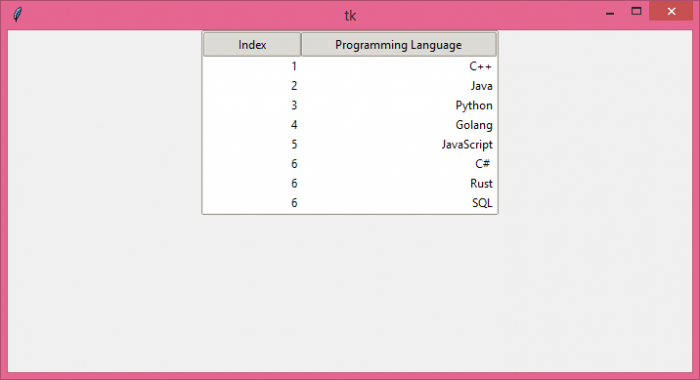# How to align text to the right in ttk Treeview widget?

TkinterPythonGUI-Programming

The Treeview widget is used to display the data in a hierarchical structure. Generally, the data is represented through the table that contains a set of rows and columns. We can add the data in the form of a table with the help of the Treeview widget.

To configure the position of the item in the column, we can use the anchor property. It sets the position of the Treeview widget column with the given value. Each row in the table is associated with a column. To align the text of the rows towards the right, we can use the anchor property and assign it the value "E", (anchor=E).

## Example

# Import the required libraries
from tkinter import *
from tkinter import ttk

# Create an instance of tkinter frame
win=Tk()

# Set the size of the tkinter window
win.geometry("700x350")

# Create an instance of Style widget
style=ttk.Style()
style.theme_use('clam')

tree.column("# 1", anchor=E, stretch=NO, width=100)
tree.column("# 2", anchor=E, stretch=NO)

# Insert the data in Treeview widget
tree.insert('', 'end',text="1",values=('1','C++'))
tree.insert('', 'end',text="2",values=('2', 'Java'))
tree.insert('', 'end',text="3",values=('3', 'Python'))
tree.insert('', 'end',text="4",values=('4', 'Golang'))
tree.insert('', 'end',text="5",values=('5', 'JavaScript'))
tree.insert('', 'end',text="6",values=('6', 'C# '))
tree.insert('', 'end',text="7",values=('6', 'Rust'))
tree.insert('', 'end',text="8",values=('6', 'SQL'))

tree.pack()

win.mainloop()

## Output

If we run the above code, it will display a table with columns having its text aligned to the right.